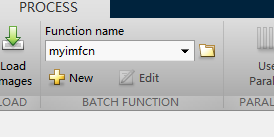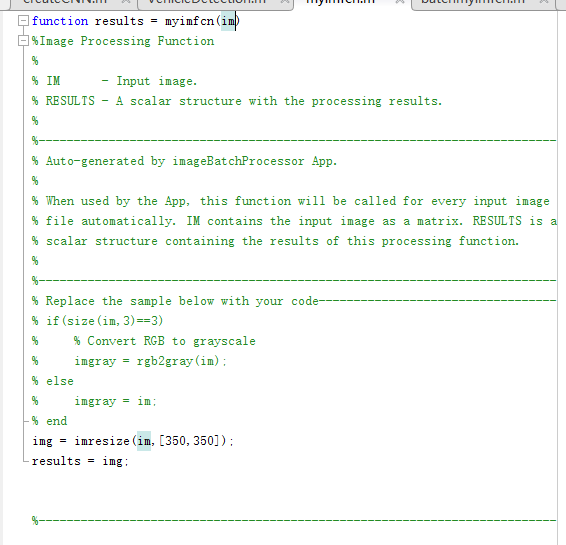2012-07-01 19:11:14 jia20003 阅读数 11303
• ###### MATLAB图像处理

全面系统的学习MATLAB在图像处理中的应用

19905 人正在学习 去看看 魏伟package com.gloomyfish.morphology;

import java.awt.Color;
import java.awt.image.BufferedImage;

public class OpeningFilter extends BinaryFilter {
private int[][] structure_element;
private Color bgColor;
private Color fgColor;

public OpeningFilter() {
structure_element = new int;
// structure_element = new int;
bgColor = Color.BLACK;
fgColor = Color.WHITE;
}

public void setBackGroundColor(Color c) {
this.bgColor = c;
}

public void setForeGroundColor(Color c) {
this.fgColor = c;
}

public void setElement(int[][] element) {
this.structure_element = element;
}

@Override
public BufferedImage filter(BufferedImage src, BufferedImage dest) {
int width = src.getWidth();
int height = src.getHeight();

if ( dest == null )
dest = createCompatibleDestImage( src, null );

int[] inPixels = new int[width*height];
int[] outPixels = new int[width*height];
src = super.filter(src, null);
getRGB( src, 0, 0, width, height, inPixels );
int index = 0;
int subrow = structure_element.length/2;
int subcol = structure_element.length/2;
int rowoffset = 0, coloffset = 0;
int index2 = 0;
for(int row=0; row<height; row++) {
int ta = 0, tr = 0, tg = 0, tb = 0;
for(int col=0; col<width; col++) {
index = row * width + col;
ta = (inPixels[index] >> 24) & 0xff;
tr = (inPixels[index] >> 16) & 0xff;
tg = (inPixels[index] >> 8) & 0xff;
tb = inPixels[index] & 0xff;
int ta2 = 0, tr2 = 0, tg2= 0, tb2 = 0;
boolean isfound = false;
for(int i=-subrow; i<=subrow; i++) {
for(int j=-subcol; j<=subcol; j++) {
rowoffset = row + i;
coloffset = col + j;
if(rowoffset >=0 && rowoffset < height) {
rowoffset = row + i;
} else {
rowoffset = 0;
}

if(coloffset >= 0 && coloffset < width) {
coloffset = col + j;
} else {
coloffset = 0;
}
index2 = rowoffset * width + coloffset;
ta2 = (inPixels[index2] >> 24) & 0xff;
tr2 = (inPixels[index2] >> 16) & 0xff;
tg2 = (inPixels[index2] >> 8) & 0xff;
tb2 = inPixels[index2] & 0xff;
if(tr2 == bgColor.getRed() && tg2 == bgColor.getGreen()) {
isfound = true;
break;
}
}
if(isfound) break;
}
rowoffset = 0;
coloffset = 0;
if(isfound) {
tr = bgColor.getRed();
tg = bgColor.getGreen();
tb = bgColor.getBlue();
outPixels[index] = (ta << 24) | (tr << 16) | (tg << 8) | tb;
} else {
outPixels[index] = (ta << 24) | (tr << 16) | (tg << 8) | tb;
}

}
}

// copy the Erosion result pixels to input pixels data
// and ready to Dilation operation
System.arraycopy(outPixels, 0, inPixels, 0, width*height);

// start to dilate the pixels data...
for(int row=0; row<height; row++) {
int ta = 0, tr = 0, tg = 0, tb = 0;
for(int col=0; col<width; col++) {
index = row * width + col;
ta = (inPixels[index] >> 24) & 0xff;
tr = (inPixels[index] >> 16) & 0xff;
tg = (inPixels[index] >> 8) & 0xff;
tb = inPixels[index] & 0xff;
int ta2 = 0, tr2 = 0, tg2= 0, tb2 = 0;
boolean isfound = false;
for(int i=-subrow; i<=subrow; i++) {
for(int j=-subcol; j<=subcol; j++) {
rowoffset = row + i;
coloffset = col + j;
if(rowoffset >=0 && rowoffset < height) {
rowoffset = row + i;
} else {
rowoffset = 0;
}

if(coloffset >= 0 && coloffset < width) {
coloffset = col + j;
} else {
coloffset = 0;
}
index2 = rowoffset * width + coloffset;
ta2 = (inPixels[index2] >> 24) & 0xff;
tr2 = (inPixels[index2] >> 16) & 0xff;
tg2 = (inPixels[index2] >> 8) & 0xff;
tb2 = inPixels[index2] & 0xff;
if(tr2 == fgColor.getRed() && tg2 == fgColor.getGreen()) {
isfound = true;
break;
}
}
if(isfound) break;
}
rowoffset = 0;
coloffset = 0;
if(isfound) {
tr = fgColor.getRed();
tg = fgColor.getGreen();
tb = fgColor.getBlue();
outPixels[index] = (ta << 24) | (tr << 16) | (tg << 8) | tb;
} else {
outPixels[index] = (ta << 24) | (tr << 16) | (tg << 8) | tb;
}

}
}

setRGB( dest, 0, 0, width, height, outPixels );
return dest;
}
}


2018-04-21 15:45:00 learning_tortosie 阅读数 10047
• ###### MATLAB图像处理

全面系统的学习MATLAB在图像处理中的应用

19905 人正在学习 去看看 魏伟

# 实验目的

1．理解并掌握形态学图像处理中的开操作和闭操作
2．熟悉并掌握MATLAB软件的使用

# 相关知识

## 1.定义

$A○B=\left(A\ominus B\right)\oplus B$

$A•B=\left(A\oplus B\right)\ominus B$

## 2.几何解释

（1）开操作的何解释
A○B的边界由B中的点建立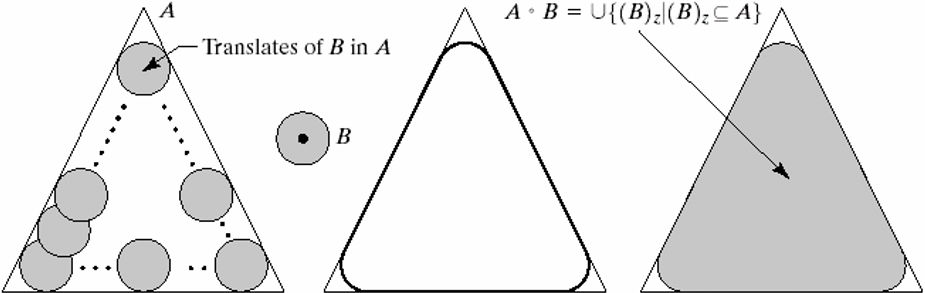（2）闭操作的几何解释
A•B的边界由B中的点建立
B在A的边界外侧滚动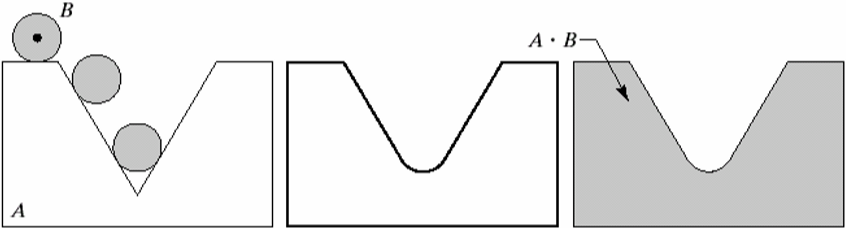## 3.相关函数说明

（1）strel

SE = STREL('arbitrary',NHOOD,HEIGHT) 创建一个指定领域的非平面结构化元素。HEIGHT是一个矩阵，大小和NHOOD相同，他指定了NHOOD中任何非零元素的高度值。
SE = STREL('ball',R,H,N) 创建一个空间椭球状的结构元素，其X-Y平面半径为R，高度为H。R必须为非负整数，H是一个实数。N必须为一个非负偶数。当N>0时此球形结构元素由一系列空间线段结构元素来近似。
SE = STREL('diamond',R) 创建一个指定大小R平面钻石形状的结构化元素。R是从结构化元素原点到其点的距离，必须为非负整数。
SE = STREL('disk',R,N) 创建一个指定半径R的平面圆盘形的结构元素。这里R必须是非负整数. N须是0, 4, 6, 8.当N大于0时,圆盘形结构元素由一组N(或N+2)个周期线结构元素来近似。当N等于0时,不使用近似，即结构元素的所有像素是由到中心像素距离小于等于R的像素组成。N可以被忽略,此时缺省值是4。注: 形态学操作在N>0情况下要快于N=0的情形。

（3）imdilate

# 实验内容

a 图是受噪声污染的指纹的二值图像，噪声为黑色背景上的亮元素和亮指纹部分的暗元素
b图是使用的结构元
c图是使用结构元素对图a腐蚀的结果：背景噪声消除了，指纹中的噪声尺寸增加
d图是使用结构元素对图c膨胀的结果：包含于指纹中的噪声分量的尺寸被减小或被完全消除，带来的问题是：在指纹纹路间产生了新的间断
e图是对图d膨胀的结果，图d的大部分间断被恢复，但指纹的线路变粗了
f图是对图e腐蚀的结果，即对图d中开操作的闭操作。最后结果消除了噪声斑点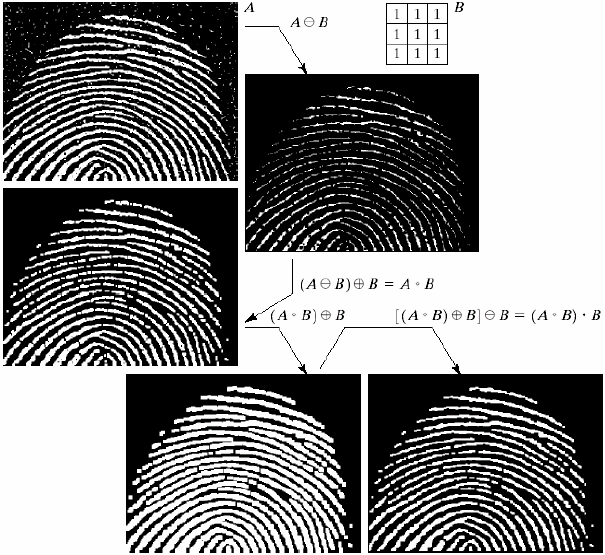# 实验结果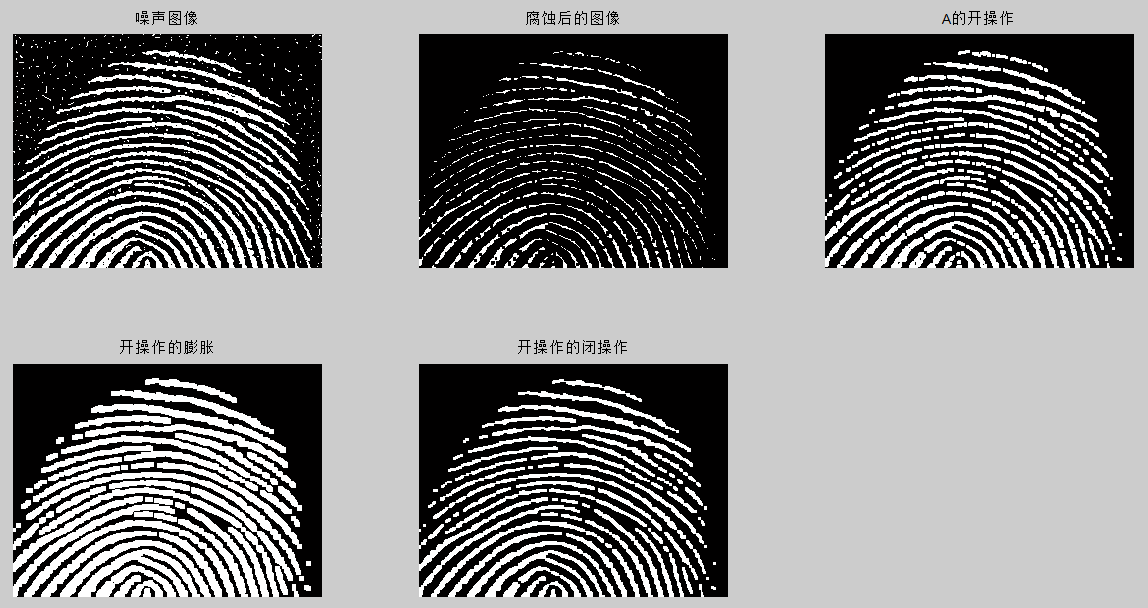# 附录

## matlab程序

A=imread('Fig0911(a)(noisy_fingerprint).tif'); %注意图片的路径要设置正确
subplot(2,3,1);
imshow(A)
title('噪声图像')
se=strel('square',3);
A2=imerode(A,se);
subplot(2,3,2);
imshow(A2)
title('腐蚀后的图像')
A3=imopen(A,se);
subplot(2,3,3);
imshow(A3)
title('A的开操作')
A4=imdilate(A3,se);
subplot(2,3,4);
imshow(A4)
title('开操作的膨胀')
A5=imclose(A3,se);
subplot(2,3,5);
imshow(A5)
title('开操作的闭操作')

2019-05-07 11:11:40 qq_34814092 阅读数 244
• ###### MATLAB图像处理

全面系统的学习MATLAB在图像处理中的应用

19905 人正在学习 去看看 魏伟

### Java OpenCV-4.0.0 图像处理07.2 图像形态学操作 图像顶帽和黑帽

Java OpenCV-4.0.0 图像处理 图像形态学操作 图像顶帽和黑帽

package com.xu.opencv.image;

import org.opencv.core.Core;
import org.opencv.core.Mat;
import org.opencv.core.Point;
import org.opencv.core.Size;
import org.opencv.highgui.HighGui;
import org.opencv.imgcodecs.Imgcodecs;
import org.opencv.imgproc.Imgproc;

/**
*
* @Title: Image.java
* @Description: OpenCV-4.0.0 图像形态学操作
* @Package com.xu.image
* @author: xuhyacinth
* @date: 2019年10月22日 12:16:55
* @version: V-1.0.0
*
*/
public class Image {

static {
}

public static void main(String[] args) {
topHat();
}

/**
* OpenCV-4.0.0 图像形态学 顶帽 (原图像与开操作之间的差值图像)
* @return: void
* @date: 2019年10月22日 12:16:55
*/
public static void topHat() {
Mat image = new Mat(src.size(), src.type());
//Imgproc.getStructuringElement 获取图片形态结构调整Size(,)的大小来去除污点
Mat kernel = Imgproc.getStructuringElement(Imgproc.MORPH_RECT, new Size(15, 15), new Point(-1, -1));
Imgproc.morphologyEx(src, image, Imgproc.MORPH_TOPHAT, kernel);
//Imgproc.resize(image, image, new Size(image.width()/3,image.height()/3));
HighGui.imshow("图像形态学 顶帽 (原图像与开操作之间的差值图像)", image);
HighGui.waitKey(1);
}

/**
* OpenCV-4.0.0 图像形态学 黑帽 (原图像与闭操作之间的差值图像)
* @return: void
* @date: 2019年10月22日 12:16:55
*/
public static void blackHat() {
Mat image = new Mat(src.size(), src.type());
//Imgproc.getStructuringElement 获取图片形态结构元素,调整Size(,)的大小来去除污点
Mat kernel = Imgproc.getStructuringElement(Imgproc.MORPH_RECT, new Size(15, 15), new Point(-1, -1));
Imgproc.morphologyEx(src, image, Imgproc.MORPH_BLACKHAT, kernel);
//Imgproc.resize(image, image, new Size(image.width()/3,image.height()/3));
HighGui.imshow("图像形态学 黑帽 (原图像与闭操作之间的差值图像)", image);
HighGui.waitKey(1);
}

}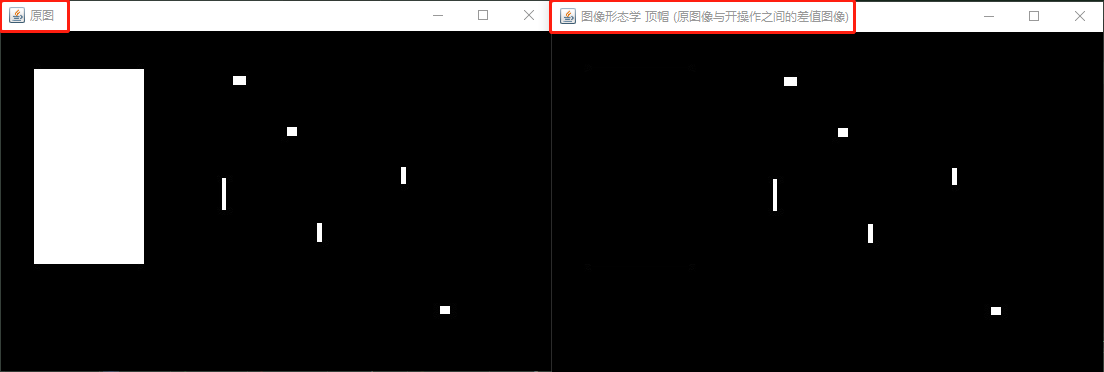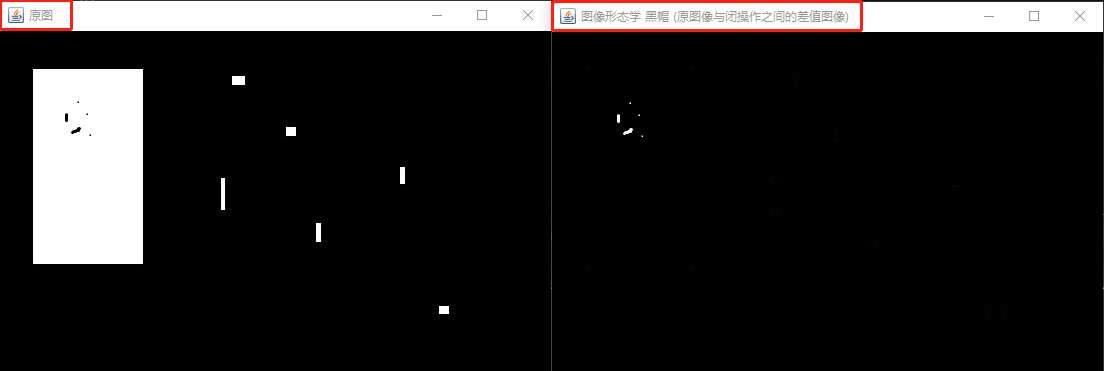2017-05-29 22:17:31 yi_tech_blog 阅读数 6158
• ###### MATLAB图像处理

全面系统的学习MATLAB在图像处理中的应用

19905 人正在学习 去看看 魏伟C++实现：把前两篇博文中的腐蚀操作和膨胀操作的代码合在一起就完成了开操作的C++实现。2019-01-16 19:58:23 ewqapple 阅读数 1649
• ###### MATLAB图像处理

全面系统的学习MATLAB在图像处理中的应用

19905 人正在学习 去看看 魏伟

matlab 提供了开箱必备的很多图像处理的工具与环境。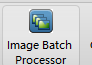图片批处理操作。打开这个工具箱，然后定制脚本